# Inelastic vibrational dynamics of CS in collision with H2 using a full-dimensional potential energy surface†

Benhui Yang*a, P. Zhang b, C. Quc, P. C. Stancil a, J. M. Bowmanc, N. Balakrishnan d and R. C. Forrey e
aDepartment of Physics and Astronomy and Center for Simulational Physics, University of Georgia, Athens, GA 30602, USA. E-mail: yang@physast.uga.edu
bDepartment of Chemistry, Duke University, Durham, NC 27708, USA
cDepartment of Chemistry, Emory University, Atlanta, GA 30322, USA
dDepartment of Chemistry and Biochemistry, University of Nevada, Las Vegas, NV 89154, USA
eDepartment of Physics, Penn State University, Berks Campus, Reading, PA 19610, USA

Received 14th September 2018 , Accepted 30th October 2018

First published on 31st October 2018

We report a six-dimensional (6D) potential energy surface (PES) for the CS–H2 system computed using high-level electronic structure theory and fitted using a hybrid invariant polynomial method. Full-dimensional quantum close-coupling scattering calculations have been carried out using this potential for rotational and, for the first time, vibrational quenching transitions of CS induced by H2. State-to-state cross sections and rate coefficients for rotational transitions in CS from rotational levels j1 = 0–5 in the ground vibrational state are compared with previous theoretical results obtained using a rigid-rotor approximation. For vibrational quenching, state-to-state and total cross sections and rate coefficients were calculated for the vibrational transitions in CS(v1 = 1,j1) + H2(v2 = 0,j2) → CS(v1′ = 0,j1′) + H2(v2′ = 0,j2′) collisions, for j1 = 0–5. Cross sections for collision energies in the range 1 to 3000 cm−1 and rate coefficients in the temperature range of 5 to 600 K are obtained for both para-H2 (j2 = 0) and ortho-H2 (j2 = 1) collision partners. Application of the computed results in astrophysics is also discussed.

## 1 Introduction

Quantum mechanical calculations of molecular scattering are of great interest for chemical dynamics and astrophysics studies.1 Carbon monosulfide (CS), the sulfur analogue of carbon monoxide, was first detected by Penzias et al.2 through the line emission of J = 3–2 in four sources including Orion A, W51, IRC + 10216, and DR 21. It is also the first sulfur-containing molecule observed in interstellar space. Since then numerous observations of CS have been reported3–10 in a variety interstellar regions. Observations of the CS emission lines are very useful for investigation of the molecular gas and its composition. An accurate prediction of the abundance of CS in the interstellar medium requires its collisional rate coefficients with ambient species. So far available collisional rate coefficients are limited to rigid-rotor calculations for a small range of rotational transitions in the vibrational ground state.11–14 In interstellar clouds, molecular hydrogen is the most abundant species and the dominant collision partner. Therefore, the vibrational and rotational rate coefficients of CS by H2 impact is of great importance in astrophysics, astrochemistry, and in interstellar regions where non-equilibrium process plays a dominant role. In particular, with the detection of CS in vibrationally excited states, the rovibrational rate coefficients are needed to analyze CS lines and model the emission and thermal balance in the interstellar medium.

Due to its astrophysical importance, there have been many theoretical studies of pure rotational cross sections and rate coefficients for CS in collision with H2. Green and Chapman11 calculated CS–H2 rotational excitation rate coefficients among first 13 rotational states of CS. Later Turner et al.12 extended the calculations to rotational levels through j1 = 20 for rate coefficient below 300 K. However these rate coefficients were computed using a CS–He PES obtained from an electron gas model.15 Vastel et al.10 performed local thermodynamic equilibrium (LTE) and non-LTE radiative transfer modeling using the NAUTILUS chemical code for the sulfur chemistry in the L1544 pre-stellar core; the rate coefficients of CS with para-H2 calculated by Green and Chapman11 were adopted to obtain the column density of CS. Albrecht presented approximate rate coefficients for CS–H2 (j2 = 0) using an empirical analytical expression16 and a fit to the rate coefficients of Green and Chapman.11 This approximation was also adopted to derive the collisional rate coefficients of linear molecules SiO, HCN, H2O.17,18 Recently, a four-dimensional (4D) potential energy surface (PES) for CS–H2 was constructed by Denis-Alpizar et al.20 based on ab initio calculations within the coupled-cluster singles and doubles plus perturbative triples[CCSD(T)] method and the augmented correlation consistent polarized valence quadruple zeta (aug-cc-pVQZ) basis set. The interaction energy between CS and H2 was obtained by subtracting the sum of the monomer potentials of CS and H2 from the total energy of the CS–H2 complex, where CS and H2 were treated as rigid rotors with the bond length of H2 fixed at its vibrationally averaged value, r0 = 1.4467a0 in the vibrational ground state and the bond length of CS fixed at its equilibrium value (re = 2.9006a0). The PES was fitted with a least squares procedure for the angular terms and interpolation of the radial coefficients with cubic splines.19 This 4D rigid rotor PES was applied to compute the rotational (de)excitation rate coefficients for the first 30 rotational levels of CS by collision with para-H2 (j2 = 0) and ortho-H2 (j2 = 1).13,14 Additionally, the CS–para-H2 rate coefficients obtained by mass-scaling the CS–He results of Lique et al.21 are also available in the Leiden Atomic and Molecular Database.23 These scaled results were also adopted by Neufeld et al.8 in their turbulent dissipation region models to determine the equilibrium level populations of CS in diffuse molecular clouds, where CS j1 = 2–1 line was observed using the IRAM 30 m telescope. For CS vibrational excitation, Lique and Spielfiedel22 performed the scattering calculations of rovibrational excitation cross sections and rate coefficients of CS by He. However, to our knowledge, there are no theoretical results available for vibrational excitation of CS with H2. The only available measurements are for the removal rate constants for vibrationally excited CS(v1 = 1) with H2, reported about a half century ago.24,25

Quantum close-coupling (CC) calculations are the primary source of rate coefficients for astrophysical modeling. Full-dimensional quantum CC formalism has been developed26,27 for collisions involving two diatomic molecules. With the development of the quantum CC scattering code TwoBC,28 diatom–diatom systems in full-dimensionality can be treated and was first used for CC calculations of rovibrational collisions of H2 with H2.29–32 Rovibrational CC calculations were also performed for the collisional systems CO–H2,33,34 CN–H2,35 SiO–H2,36 along with coupled-states approximation calculations in 5D and 6D.37–39

Here we report the first full-dimensional PES and the first vibrational inelastic scattering calculations for the CS–H2 system. The paper is organized as follows. A brief description of the Theoretical methods for the electronic structure and scattering calculations are given in Section 2. The results are presented and discussed in Section 3 followed by Astrophysical applications in Section 4. Section 5 summarizes the results and presents an outlook on future work.

## 2 Theoretical methods

We briefly describe in this section the theoretical methods applied in the computation and analytical fit of the CS–H2 PES as well as the quantum CC calculations in full dimensionality. The reader is referred to ref. 30, 33 and 40 for the details of the theoretical methodology.

### 2.1 Potential energy surface computation and fit

The interaction potential of CS–H2 in the electronic ground state was computed on a 6D grid using Jacobi coordinates (R,r1,r2,θ1,θ2,ϕ). As shown in Fig. 1, R denotes the distance between the centers of mass of CS and H2, and r1 and r2 are the bond lengths describing the vibration of CS and H2, respectively. The angles θ1 and θ2, respectively, are the in-plane orientation angles of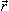1 and2 with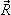, while ϕ denotes the out-of-plane dihedral angle. The PES was computed with the MOLPRO suite of computational chemistry codes.41,42 The intermolecular distance R was chosen in the range of 4.5–25a0 and the bond distances are confined to 2.55 ≤ r1 ≤ 3.51a0 and 1.05 ≤ r2 ≤ 2.27a0. Here a0 = 0.529 Å is the Bohr radius. To produce an accurate 6D PES with proper symmetry the ab initio calculations were performed over the angular coordinates 0 ≤ θ1 ≤ 360° and 0 ≤ θ2, ϕ ≤ 180°, where θ1 = θ2 = 0° corresponds to the collinear configuration C–S–H–H.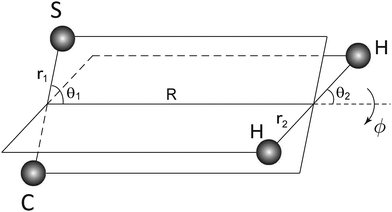Fig. 1 The six-dimensional Jacobi coordinates for the CS–H2 system.

The ab initio electronic structure computations of potential energies were performed using the explicitly correlated coupled-cluster (CCSD(T)-F12b) method.43,44 All the calculations employed aug-cc-pV5Z (for H and C atoms)45 and aug-cc-pV(5+d)Z (for S atom) orbital basis set,46 and the corresponding MP2FIT (for H and C atoms) and aug-cc-pwCV5Z (for S atom) auxiliary bases47,48 for density fitting. The aug-cc-pV6Z-RI auxiliary bases (without k functions)49 were used for the resolutions of the identify and density-fitted Fock matrices for all orbital bases. No scaled triples correction was used in the CCSD(T)-F12 calculation. The interaction PES was corrected for basis set superposition error (BSSE)50 using the counter-poise (CP)51 method. Benchmark calculations at this CCSD(T)-F12 level were carried out on selected molecular configurations and results were compared with those from the conventional CCSD(T) method using aug-cc-pV5Z. The CP corrected interaction energy agrees closely with those derived from CCSD(T)/aug-cc-pV5Z.

The full-dimensional CS–H2 interaction potential, referred to as VCSH2, is a hybrid one combining a fit to the full ab initio data set (denoted VI) and a fit to the long-range data (denoted VII) and is expressed by

 V = (1 − s)VI + sVII, (1)
the switching function s is given as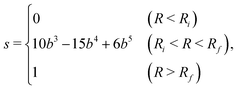(2)
where b = (RRi)/(RfRi), Ri = 8.5a0 and Rf = 10.5a0 are the lower and upper bound of the switching region, respectively. Both VI and VII are expanded in the form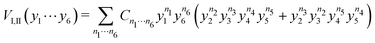(3)
and have been fitted in 6D using an invariant polynomial method,40,52 where yi = exp(−di/p) are Morse-type variables and p is a user-specified parameter with p = 2.0a0 for VI and p = 7.0a0 for VII. The parameters di are the internuclear distances between two atoms and defined as d1 = dCS, d2 = dCH, d3 = dCH, d4 = dSH, d5 = dSH and d6 = dHH. The powers n1,⋯, n6 satisfy n1 +⋯+ n6 ≤ 7 and n2 + n3 + n4 + n5 ≠ 0, and the interaction potential is guaranteed to approach zero when CS and H2 are infinitely far apart for all r1 and r2. The total number of fitted linear coefficients Cn1⋯n6 is 882, and these coefficients were determined via linear least-squares fitting using the software MSA.53 The root mean square (RMS) error in the long range fit VII is 0.062 cm−1, for VI the RMS error is 14.2 cm−1.

In Fig. 2 the R dependence of VCSH2 PES is compared with the PES of Denis-Alpizar et al.20 for (θ1, θ2, ϕ) = (0°, 0°, 0°), (180°, 0°, 0°), (180°, 90°, 0°), and (90°, 90°, 90°). Good agreement between our PES and the PES of Denis-Alpizar et al.20 is displayed, except that our PES is a little shallower at the global minimum. Our fitted PES has a depth of −162.56 cm−1 at the collinear structure with the C atom towards to H2 and R = 8.6a0. The minimum of the PES of Denis-Alpizar et al.20 is about −173 cm−1 and also corresponds to a collinear geometry with same R value. Fig. 3 shows a two-dimensional contour plot of the VCSH2 PES in θ1, θ2 space for fixed values of r1 = 2.9006a0, r2 = 1.4011a0, R = 8.5a0, and ϕ = 0°.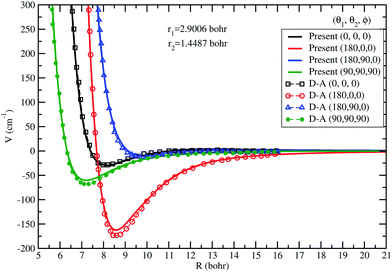Fig. 2 The R dependence of the CS–H2 interaction potential, VCSH2 for (θ1, θ2, ϕ) = (0°, 0°, 0°), (180°, 90°, 0°), (90°, 90°, 90°), and (180°, 0°, 0°). The bond lengths of CS and H2 are fixed at equilibrium value and vibrationally averaged value in the rovibrational ground state, respectively. Symbols are for the PES of Denis-Alpizar et al.20 (D–A).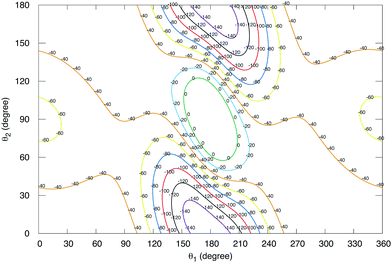Fig. 3 Contour plots of the potential VCSH2 as a function of θ1 and θ2 for r1 = 2.9006a0, r2 = 1.4011a0, R = 8.5a0, and ϕ = 0°.

### 2.2 Scattering calculation

The full-dimensional quantum CC scattering calculations were performed using the TwoBC code.28 To facilitate the scattering calculations, it is convenient to expand the angular dependence of the interaction potential between CS and H2, V(1,2,) at each intermolecular distance R according to,54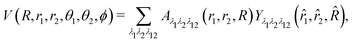(4)
where the bi-spherical harmonic function is expressed as,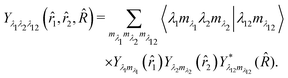(5)
Terms 0 ≤ λ1 ≤ 8 and 0 ≤ λ2 ≤ 4 are retained in the expansion. Only even values of λ2 contribute because H2 is homonuclear.

We use a combined molecular state (CMS)30 notation, (v1j1v2j2), to describe a combination of rovibrational states for CS(v1j1) and H2(v2j2), where ji and vi (i = 1, 2) are the rotational and vibrational quantum numbers of CS and H2. For a rovibrational transition the state-to-state cross section can be obtained as a function of the collision energy Ec from the corresponding scattering matrix S: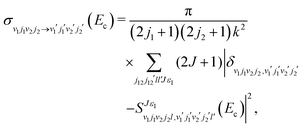(6)
where the wave vector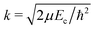and the primed quantities refer to the final state. The quantum number J denotes the total angular momentum,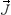=+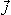12, with12 =1 +2 and l is the orbital angular momentum quantum number.

The total quenching cross section of CS from initial state (v1j1v2j2) → (v1′;v2j2′) is obtained by summing the state-to-state quenching cross sections over the final rotational state of j1′ of CS in vibrational state v1′,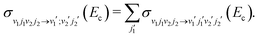(7)

From the state-to-state cross section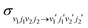the corresponding rate coefficient at a temperature T can be obtained by averaging it over a Boltzmann distribution of collision energies.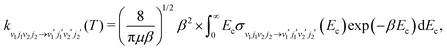(8)
where μ is the reduced mass of the CS–H2 system, β = (kBT)−1, and kB is Boltzmann's constant.

In the full-dimensional rovibrational scattering calculations with the TwoBC code28 the log-derivative matrix propagation method of Johnson55 was employed to propagate the CC equations from R = 4.5a0 to 25a0. The number of Gauss–Hermite quadrature points Nr1, Nr2; the number of Gauss–Legendre quadrature points in θ1 and θ2, Nθ1, Nθ2; and the number of Chebyshev quadrature points in ϕ, Nϕ adopted to project out the expansion coefficients of the PES are listed in Table 1. In the scattering calculations, the monomer potentials of Paulose et al.56 and Schwenke57 are used to describe the rovibrational motions of CS and H2, respectively.

Table 1 Parameters used in the scattering calculations
Basis set N θ 1 (Nθ2) N ϕ N r 1 (Nr2) λ 1 λ 2
a Basis set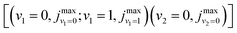is presented by the maximum rotational quantum number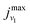and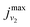included in each relevant vibrational level v1 and v2 for CS and H2, respectively. b Maximum values of the total angular momentum quantum number J(JE1,JE2,JE3,JE4) used in scattering calculations for collision energies E1 = 10, E2 = 100, E3 = 1000, and E4 = 3000 cm−1, respectively.
6D rotation
para-H2–CS j max1 = 30, j2 = 0,2 12 8 18 8 4 (16, 30, 80, 160)b
ortho-H2–CS j max1 = 30, j2 = 1,3 12 8 18 8 4 (18, 32, 82, 162)b
6D rovibration
para-H2–CS [(0,35;1,20)(0,2)]a 12 8 18 8 4 (16, 30, 80, 160)b
ortho-H2–CS [(0,35;1,20)(0,3)]a 12 8 18 8 4 (18, 32, 82, 162)b

## 3 Results and discussion

### 3.1 Pure rotational de-excitation

The rotational de-excitation cross sections of CS(v1 = 0, j1) in collision with H2 have been calculated for the transitions, CS(v1 = 0, j1) + H2(v2 = 0, j2) → CS(v1′ = 0, j1′) + H2(v2′ = 0, j2′), where both CS and H2 are taken to be in their ground vibrational states, i.e., (0j10j2) → (0j1′0j2′). We performed the calculations in full-dimension for the initial rotational states of CS j1 = 1–5, with collision energy ranging from 1 to 3000 cm−1. Sufficient number of partial waves were included to ensure the convergence of the cross sections. Convergence tests were also made with respect to the para-H2 basis, it was found that the effect from inclusion of the j2 = 4 state is generally less than 1%. The basis sets and partial waves used in the scattering calculations are provided in Table 1. In Fig. 4 the state-to-state quenching cross sections of CS from initial state j1 = 5 from our calculations are compared with the results of Denis-Alpizar et al.13 Results are presented for both para-H2 (j2 = 0) and ortho-H2 (j2 = 1) for final states j1′ = 0, 1, 2, 3, and 4. Fig. 4(a) shows that for para-H2, for collision energies below ∼30 cm−1, all state-to-state cross sections display resonances due to the decay of quasibound states supported by the van der Waals well of the PES. At collision energies below 200 cm−1, the cross sections generally increase with increasing j1′ with the smallest cross section corresponding to the j1 = 5 → 0 transition, i.e., the largest |Δj1 = j1′ − j1|. While for collision energies above 200 cm−1 the state-to-state quenching cross sections are dominated by Δj1 = −2 transitions. Above ∼1000 cm−1, the cross sections for j1 = 5 → 1 becomes larger than j1 = 5 → 2. The agreement between our results and the results of Denis-Alpizar et al.13 is reasonable, though differences are seen in the low collision energy region due to the presence of scattering resonances. These differences tend to disappear as the collision energy is increased. One exception is the transition j1 = 5 → 0, where good agreement is only obtained for collision energies between 150 and 500 cm−1, but these cross sections have the smallest magnitude. For ortho-H2, as Fig. 4(b) depicts, the state-to-state cross sections exhibit similar trend as for para-H2. The energy gap law is more pronounced here with a decrease of the cross section with increase in |Δj1|. However, the resonance features are almost absent, presumably due to the broader range of partial waves involved. The quenching cross sections are dominated by Δj1 = −1 transition for collision energies below ∼1000 cm−1, followed by Δj1 = −2. Except for the j1 = 5 → 0 transition at low collision energies, our results are in very good agreement with the results of Denis-Alpizar et al.13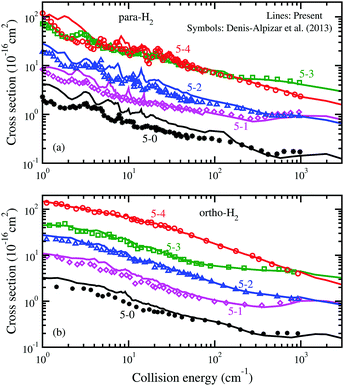Fig. 4 Rotational state-to-state de-excitation cross sections for CS(j1) + H2(j2) → CS(j1′) + H2(j2′), j1 = 5, j1′ < j1. Lines are for the present results and symbols are for the results of Denis-Alpizar et al.13 (a) CS in collision with para-H2, j2 = j2′ = 0; (b) CS in collision with ortho-H2, j2 = j2′ = 1.

Fig. 5(a and b) display the total rotational quenching cross sections from initial states j1 = 1–5 of CS with para-H2 (j2 = 0) and ortho-H2 (j2 = 1), respectively. It is found that for both para-H2 and ortho-H2, except for collision energies below ∼ 3 cm−1, the total rotational quenching cross sections increase with increasing initial j1. For para-H2, the resonance features observed in the state-to-state cross sections can also be noted in the total quenching cross sections, particularly in the j1 = 1 state.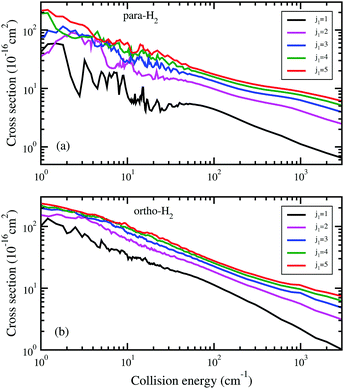Fig. 5 Total rotational de-excitation cross sections for CS(j1) from initial states j1 = 1, 2, 3, 4, and 5. (a) CS in collision with para-H2, j2 = j2′ = 0; (b) CS in collision with ortho-H2, j2 = j2′ = 1.

In Fig. 6, we provide a comparison between the para-H2 and ortho-H2 cross sections for initial rotational states j1 = 1 and 2. For Δj1 = −1, the cross section of ortho-H2 are larger than that of para-H2 over the whole range of collision energy. While for the j1 = 2 → 0 transition, the cross sections of para-H2 and ortho-H2 are of comparable magnitude, though the resonance dominated region for para-H2 shows significant differences.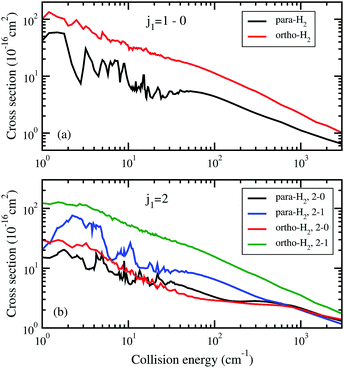Fig. 6 Comparison of the rotational state-to-state de-excitation cross sections for CS in collision with para- and ortho-H2. (a) From initial state j1 = 1; (b) from initial state j1 = 2.

In Fig. 7(a and b) we show the temperature dependence of the rate coefficients for rotational quenching of CS from j1 = 2 induced by para- and ortho-H2 collisions, respectively, in the temperatures range of 5 to 600 K. For comparison, the corresponding rate coefficients of Denis-Alpizar et al.14 are also included. The temperature dependence is the steepest for the j1 = 2 → 0 rate coefficient for para-H2 collisions. While for j1 = 2 → 1, the rate coefficient first decreases with temperature from T = 5–20 K, then increases gradually with increasing temperature. Compared to the results of Denis-Alpizar et al.,14 good agreement is observed for the j1 = 2 → 0 rate coefficients. However, for the j1 = 2 → 1 transition, our rate coefficient is larger than the results of Denis-Alpizar et al. but the differences tend to vanish as the temperature is increased. As shown in Fig. 2, the VCSH2 PES and the PES of Denis-Alpizar et al.20 have different well depths with VCSH2 being the shallower one. This leads to different resonance structures in the cross sections on the two PESs, particularly, at low collision energies. The differences in the rate coefficients at low temperatures may be attributed to the different resonance structures. Fig. 8 provides a comparison of the state-to-state rate coefficients from the present work for j1 = 5 with that of Denis-Alpizar et al..14 The para-H2 results shown in Fig. 8(a) are in reasonable agreement with the results of Denis-Alpizar et al. while the ortho-H2 results presented in Fig. 8(b) are in excellent agreement.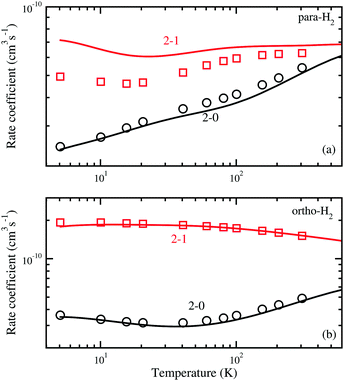Fig. 7 Rotational state-to-state de-excitation rate coefficients compared with the results of Denis-Alpizar et al. (D–A)14 for CS(j1 = 2) in collision with (a) para-H2 (j2 = 0) and (b) ortho-H2 (j2 = 1).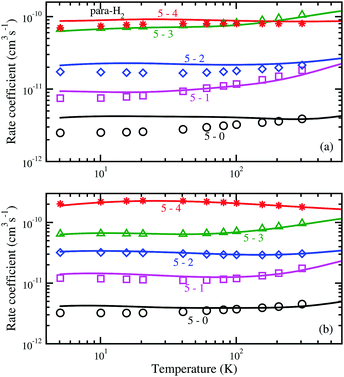Fig. 8 Rotational state-to-state de-excitation rate coefficients compared with D–A for CS(j1 = 5) in collision with (a) para-H2(j2 = 0) and (b) ortho-H2(j2 = 1).

When rate coefficients for a particular collision complex are lacking, it has been argued that rate coefficients from chemically similar systems could be at adopted.58 We test this idea by comparing the current results from CS to prior work on SiO36 and SiS.59,60 In Fig. 9, the state-to-state rate coefficients for rotational quenching from initial state j1 = 3 for para-H2 collisions are shown. The rate coefficients differ by a factor of ∼5 with no clear trend. Adoption of such an approximation is clearly not advisable.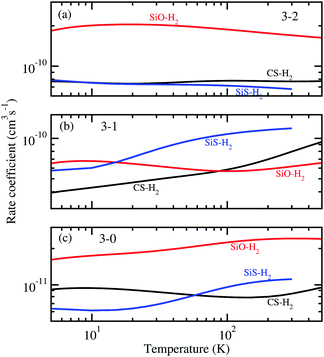Fig. 9 Rotational state-to-state de-excitation rate coefficients from initial j1 = 3 of CS, SiO, and SiS in collisions with para-H2(j2 = 0). (a) j1 = 3 → 2, (b) j1 = 3 → 1, (c) j1 = 3 → 0.

### 3.2 Vibrational quenching

Here we present close-coupling calculations of rovibrational transitions in CS due to H2 collisions in full-dimensionality, the main focus of this work.

The state-to-state quenching cross sections were computed for CS rovibrational transitions from v1 = 1, CS(v1 = 1, j1) + H2(v2 = 0, j2) → CS(v1′ = 0, j1′) + H2(v2′ = 0, j2′), i.e., (1j10j2) → (0j1′0j2′). In our calculations, initial j1 = 0–5 and final j1′ = 0, 1, 2,⋯, 35. For para-H2, j2 = 0, j2′ = 0 and 2, while for ortho-H2, j2 = 1, j2′ = 1 and 3 are considered. We only consider the rotational excitations of H2 within its ground vibrational state, i.e., v2 = v2′ = 0 for all of the results presented here. The basis sets employed in the scattering calculations are given in Table 1. All vibrational quenching cross sections were calculated for collision energies between 1 and 3000 cm−1.

As examples, we show in Fig. 10 the state-to-state quenching cross sections from initial CMS (1000) and (1001) into selected final rotational states in v1′ = 0, j1′ = 0, 2, 4, 6, 8, 10, 15, 20, and 25. These results correspond to rotationally elastic scattering of the H2 molecule, i.e., j2 = 0 → j2′ = 0. As seen from Fig. 10, for both para-H2 and ortho-H2 colliders, a large number of resonances are observed in the cross sections at collision energies between 1 and 50 cm−1. We also notice that the resonances persist to higher energies with increasing j1′. Additionally, the state-to-state quenching cross sections increase with collision energy for energies above ∼50 cm−1. Overall, the cross sections corresponding to j1′ ≤ 15 show similar resonance structures and exhibit similar energy dependence. The cross sections for j1′ = 20 and 25 are about two to three orders of magnitude smaller than other transitions for both para- and ortho-H2 for collision energies below 300 cm−1, however they increase rapidly with energy, and the cross section of j1′ = 25 becomes the largest at an energy of 3000 cm−1.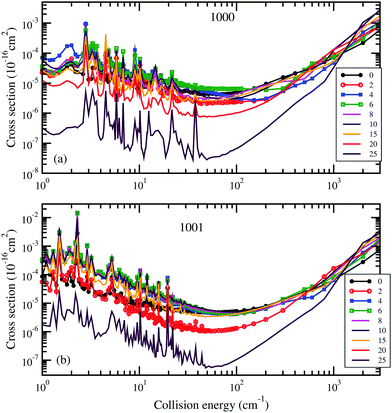Fig. 10 Vibrational state-to-state quenching cross section for transitions CS(v1 = 1, j1 = 0) + H2(j2) → CS(v1′ = 0, j1′) + H2(j2), j1′ = 0, 2, 4, 6, 8, 10, 15, 20, and 25. (a) para-H2(j2 = 0) and (b) ortho-H2(j2 = 1).

Next we discuss the effect of vibrational excitation of CS on its pure rotational quenching. This is provided in Fig. 11 for v1 = 0 and 1 for rotational levels j1 = 1 and 5 to all j1′ < j1 for para-H2 with H2 scattered elastically. It can be seen that the cross sections are nearly identical for v1 = 0 and 1, over the whole range of energies considered in this work demonstrating the negligible effect of vibrational excitation of CS on pure rotational quenching. This is due to the almost harmonic nature of the v = 0 and 1 vibrational levels of CS. Similar results are found for ortho-H2 collisions. This also means that, effect of vibrational excitation of CS can be ignored for pure rotational transitions in CS in collisions with ortho- and para-H2, at least for low vibrational levels. A similar conclusion was also drawn for the SiO–H2 system.36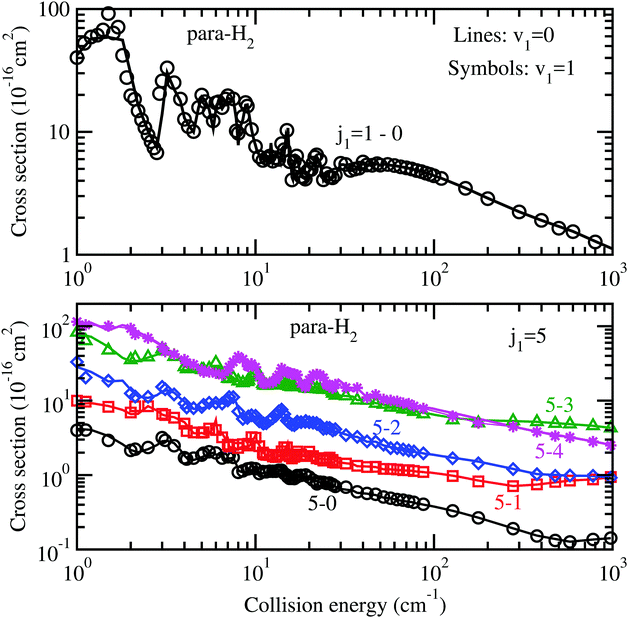Fig. 11 Rotational quenching state-to-state excitation cross section of CS from initial states j1 = 1 and 5 to j1′, j1′ < j1. (a) and (b): para-H2. For each transition, the comparison is made between v1 = 0 and v1 = 1.

Using eqn (7) the total vibrational quenching cross section of CS from initial CMS (1000) and (1001) was obtained by summing the state to state quenching cross sections over the final rotational state j1′ of CS in vibrational state v1 = 0. These total quenching cross section were calculated for elastic and inelastic transitions in H2.

Fig. 12(a) presents the energy dependence of the total v1 = 1 → v1′ = 0 quenching cross section with para-H2 from CMSs (1000) for elastic (j2 = 0 → j2′ = 0) and inelastic (j2 = 0 → j2′ = 2) transitions in H2. While they exhibit similar energy dependencies and similar resonance structures at low energies, the cross sections for elastic transition in H2 are about a factor of two larger than its inelastic counterpart in the entire energy range. For ortho-H2 and initial CMS (1001), Fig. 12(b) shows that the total vibrational quenching cross sections for elastic (j2 = 1 → j2′ = 1) and inelastic (j2 = 1 → j2′ = 3) transitions in H2 are comparable for collision energies below ∼200 cm−1. For energies above ∼200 cm−1, the cross section for H2 rotation preserving transition becomes increasingly dominant.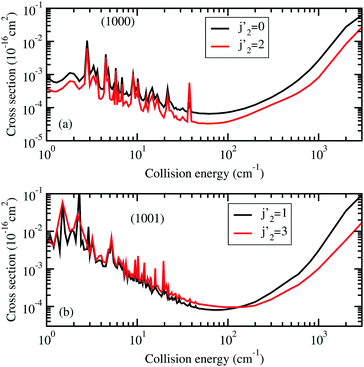Fig. 12 Total vibrational quenching cross section for transitions CS(v1 = 1, j1) + H2(j2) → CS(v1′ = 0) + H2(j2). j1 = 0. (a) para-H2 (j2 = 0). (b) ortho-H2 (j2 = 1).

We also calculated the state-to-state and total vibrational quenching rate coefficients of CS(v1 = 1, j1) in collisions with para-H2 (j2 = 0) and ortho-H2 (j2 = 1) for j1 = 0–5. State-to-state rate coefficients are obtained for j1′ = 0, 1, 2,⋯, 35 and j2′ = 0 and 2 for para-H2 and j2′ = 1 and 3 for ortho-H2. Unfortunately, there are no published experimental or theoretical state-to-state rate coefficients available for comparison. As an example, in Fig. 13 we show the total vibrational quenching rate coefficients for temperatures between 1 and 600 K for CMS (1000) to (v1′ = 0) and j2′ = 0, and CMS (1001) to (v1′ = 0) and j2′ = 1.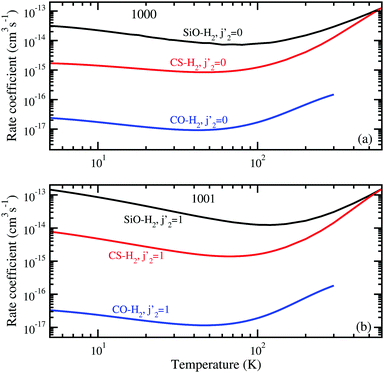Fig. 13 Total rate coefficients for the vibrational quenching of CS compared to the same transitions for CO from ref. 33 and SiO from ref. 33. (a) from (1000) to v1′ = 0 + para-H2(v2′ = 0, j2′ = 0). (b) From (1001) to v1′ = 0 + ortho-H2(v2′ = 0, j2′ = 1).

Previously we have provided a comparison of CS–H2 rate coefficients with that of CO–H2 (ref. 33) and for SiO–H2 (ref. 36). It was shown that for the same transitions, the total quenching rate coefficients of CO–H2 are typically ∼2–3 orders of magnitude smaller than that of CS–H2 and SiO–H2. The large magnitude of SiO–H2 rate coefficients are likely due to the high anisotropy of the SiO–H2 PES. Interestingly, at high temperatures the CS–H2 rate coefficients merge with those of SiO–H2. Furthermore, generally the rate coefficients increase with increasing well depth of the interaction PES, the SiO–H2 rate coefficients are largest with a well depth of 293.2 cm−1 and CO–H2 rate coefficients are smallest with a well depth of 93.1 cm−1.

## 4 Astrophysical applications

A quantitative non-LTE modeling of molecular excitation and line formation processes from infrared and submillimetre observations requires detailed knowledge of collisional rate coefficients for transitions between the rovibrational states of molecules over a wide range of levels and temperatures. Molecular collisions, which are responsible for most of the excitation and reaction processes, are important in the interstellar medium (ISM). Particularly, in cold molecular clouds, collisional processes are the dominant factor in analysis of emission and absorption spectra, and H2 and He are the most important collision partners due to their large abundances, except in photodissociation regions (PDRs) and diffuse gas where collisions with electrons and H can become important. However, direct measurements of the collisional rate coefficients are difficulty and complicated; astrophysical modeling heavily rely on theoretical calculations.61

Carbon monosulfide and its isotopic species have been detected in a large range of astrophysical environments. As a high-density tracer of dense gas, CS emission is normally used in the study of star forming regions. Using the IRAM Plateau de Bure interferometer Guilloteau et al.62 observed two CS transitions j1 = 3–2 and j1 = 5–4 in the DM Tau disk. The line intensities were used to determine the magnitude of the turbulent motions in the outer parts of the disk. Maxted et al.63 detected emission j1 = 1–0 and j1 = 2–1 of CS and its isotopologues in four of the densest cores towards the western rim of supernova remnant RX J1713.7-3946 and confirmed the presence of dense gas ≥104 cm−3 in the region. More recently, CS emission (1–0) was used in the investigation of the nature of the supernova remnant HESS J1731-347.64 Line emission from rotational transition j1 = 7–6 within the vibrationally excited state v = 1 was observed in IRAS 16293-2422.65 Gómez-Ruiz et al.66 detected CS and isotopologues 12C32S, 12C34S, 13C32S, 12C33S, for a total of 18 transitions with Herschel/HIFI and IRAM-30m at L1157-B1. With Herschel/HIFI spectral line survey67 CS transitions were detected toward the Orion Bar, tracing the warm and dense gas with temperatures of 100–150 K and densities of 105–106 cm−3. NLTE analysis was also performed with RADEX, where the CS–H2 rate coefficients were obtained by reduced-mass scaling the rate coefficients with He of Lique et al.21 Using the IRAM 30 m telescope, Aladro et al.68 performed a molecular line survey towards the circumnuclear regions of eight active galaxies, CS and its isotopologues were identified in M83, M82, M51, and NGC 253. Using the Atacama Large Millimeter/submillimeter Array (ALMA), Takano et al.69 performed a high resolution imaging study of molecular lines near the supermassive black hole at the center of galaxy NGC 1068. CS emission of j1 = 2–1 was distributed both in the circumnuclear disk and the starburst ring. Walter et al.70 investigated dense molecular gas tracers in the nearby starburst galaxy NGC 253 using ALMA, CS(j1 = 2–1) was detected in the molecular outflow.

Vibrationally excited molecules, which are excited by collision or infrared radiation, can be used to probe extreme physical conditions with high gas densities and temperatures. The first observation of vibrationally excited CS in the circumstellar shell of IRC + 10216 was reported by Turner71 through transitions j1 = 2 → 1 and 5 → 4 in v1 = 1. In 2000, Highberger et al.72 reobserved these lines and also detected new transitions of j1 = 3–2, 6–5, and 7–6 of vibrationally excited CS(v = 1) toward IRC + 10216. Using Submillimeter Array, Patel et al.73 detected the CS v1 = 2, j1 = 7–6 transition from the inner envelope of IRC + 10216. Finally, vibrational absorption lines for CS have been detected for its fundamental band near 8 μm in IRC + 10216.74 These observations used the Texas Echelon-cross-Eschelle Spectrograph (TEXES) on the 3 m Infrared Telescope facility.

Our present full-dimensional scattering calculation will be able to provide accurate rovibrational state-to-state CS–H2 collisional data for future modeling of protostars, the infrared sources discussed above, and future FIR and submillimeter observations with Herschel and ALMA. Furthermore, CS vibrational bands in the 1–5 μm region will be accessible by the James Webb Space Telescope (JWST) to be launched in 2021 and currently with SOFIA using the EXES (5–28 μm) or FORCAST (5–40 μm) instruments. In PPDs, CS vibrational lines probe the inner warm regions which are exposed to the UV radiation from the protostar.

## 5 Summary

We constructed a 6-dimensional potential energy surface for CS–H2 based on high-level electronic structure calculations. State-to-state cross sections for rotational and rovibrational transitions in CS in collisions with H2 are reported using full-dimensional quantum scattering calculations and an analytical fit of the potential surface.

Cross sections and rate coefficients for pure rotational transitions from the present study are found to be in good agreement with the rigid-rotor approximation calculations of Denis-Alpizar et al.13,14 using a 4-dimensional potential surface. The vibrational quenching cross sections and rate coefficients have been reported for the first time. In future work, we plan to extend the current calculations to higher rotational and vibrational states of CS and include the effect of vibrational excitation of the H2 molecule.

## Conflicts of interest

There are no conflicts of interest to declare.

## Acknowledgements

Work at UGA and Emory was supported by NASA grant NNX16AF09G, at UNLV by NSF Grant No. PHY-1505557, and at Penn State by NSF Grant No. PHY-1503615. This study was supported in part by resources and technical expertise from the UGA Georgia Advanced Computing Resource Center (GACRC), and UNLV National Supercomputing Institute & Dedicated Research Network. We thank Shan-Ho Tsai (GACRC), Jeff Deroshia (UGA Department of Physics and Astronomy), and Ron Young (UNLV) for computational assistance.

## References

1. D. Flower, Molecular Collisions in the Interstellar Medium, Cambridge University Press, Cambrdige, 2nd edn, 2011 Search PubMed.
2. A. A. Penzias, P. M. Solomon, R. W. Wilson and K. B. Jefferts, Astrophys. J., 1971, 168, L53–L58 CrossRef CAS.
3. R. W. Wilson, A. A. Penzias, P. G. Wannier and R. A. Linke, Astrophys. J., 1976, 204, L135–L137 CrossRef CAS.
4. K. S. K. Swamy, Astron. Astrophys., 1981, 97, 110 CAS.
5. H. A. Thronson Jr. and C. J. Lada, Astrophys. J., 1984, 284, 135 CrossRef.
6. K. S. Noll, M. A. McGrath, L. M. Trafton, S. K. Atreya, J. J. Caldwell, H. A. Weaver, R. V. Yelle, C. Barnet and S. Edgington, Science, 1995, 267, 1307–1313 CrossRef CAS PubMed.
7. D. P. Ruffle, T. W. Hartquist, P. Caselli and D. A. Williams, Mon. Not. R. Astron. Soc., 1999, 306, 691–695 CrossRef CAS.
8. D. A. Neufeld, B. Godard and M. Gerin, et al. , Astron. Astrophys., 2015, 577, A49 CrossRef.
9. R. Moreno, E. Lellouch and T. Cavalie, et al. , Astron. Astrophys., 2017, 608, L5 CrossRef.
10. C. Vastel, D. Quénard and R. Le Gal, et al. , Mon. Not. R. Astron. Soc., 2018, 478, 5519–5537 CrossRef.
11. S. Green and S. Chapmen, Astrophys. J., Suppl. Ser., 1978, 37, 169–194 CrossRef CAS.
12. B. E. Turner, K.-W. Chan, S. Green and D. A. Lubowich, Astrophys. J., 1992, 399, 114–133 CrossRef CAS.
13. O. Denis-Alpizar, T. Stoecklin, P. Halvick and M.-L. Dubernet, J. Chem. Phys., 2013, 139, 204304 CrossRef PubMed.
14. O. Denis-Alpizar, T. Stoecklin, S. Guilloteau and A. Dutrey, Mon. Not. R. Astron. Soc., 2018, 478, 1811–1817 CrossRef.
15. R. G. Gordon and J. S. Kim, J. Chem. Phys., 1972, 56, 3122–3133 CrossRef CAS.
16. M. A. Albrecht, Astron. Astrophys., 1983, 127, 409–410 CAS.
17. J. H. Bieging, L. B. G. Knee, W. B. Latter and H. Olofsson, Astron. Astrophys., 1998, 339, 811–821 CAS.
18. K. T. Wong, T. Kamiński, K. M. Menten and F. Wyrowski, Astron. Astrophys., 2016, 590, A127 CrossRef.
19. G. Guillon, T. Stoecklin, A. Voronin and P. Halvick, J. Chem. Phys., 2008, 129, 104308 CrossRef CAS PubMed.
20. O. Denis-Alpizar, T. Stoecklin, P. Halvick, M.-L. Dubernet and S. Marinakis, J. Chem. Phys., 2012, 137, 234301 CrossRef PubMed.
21. F. Lique, A. Spielfiedel and J. Cernicharo, Astron. Astrophys., 2006, 451, 1125–1132 CrossRef CAS.
22. F. Lique and A. Spielfiedel, Astron. Astrophys., 2007, 451, 1179–1185 CrossRef.
23. F. L. Schöier, F. F. S. van der Tak, E. F. van Dishoeck and J. H. Black, Astron. Astrophys., 2005, 432, 369–379 CrossRef.
24. I. W. M. Smith, Trans. Faraday Soc., 1968, 64, 3183–3191 RSC.
25. C. Morley and I. W. M. Smith, Trans. Faraday Soc., 1971, 67, 2575–2585 RSC.
26. K. Takayanagi, Adv. At. Mol. Phys., 1965, 1, 149–194 Search PubMed.
27. S. K. Pogrebnya and D. C. Clary, Chem. Phys. Lett., 2002, 363, 523–528 CrossRef CAS.
28. R. V. Krems, TwoBC – quantum scattering program, University of British Columbia, Vancouver, Canada, 2006 Search PubMed.
29. G. Quéméner, N. Balakrishnan and R. V. Krems, Phys. Rev. A: At., Mol., Opt. Phys., 2008, 77, 030704 CrossRef.
30. G. Quéméner and N. Balakrishnan, J. Chem. Phys., 2009, 130, 114303 CrossRef PubMed.
31. S. F. dos Santos, N. Balakrishnan, S. Lepp, G. Quéméner, R. C. Forrey, R. J. Hinde and P. C. Stancil, J. Chem. Phys., 2011, 134, 214303 CrossRef PubMed.
32. S. F. dos Santos, N. Balakrishnan, R. C. Forrey and P. C. Stancil, J. Chem. Phys., 2013, 138, 104302 CrossRef PubMed.
33. B. H. Yang, P. Zhang, X. Wang, P. C. Stancil, J. M. Bowman, N. Balakrishnan and R. C. Forrey, Nat. Commun., 2015, 6, 6629 CrossRef CAS PubMed.
34. B. H. Yang, N. Balakrishnan, P. Zhang, X. Wang, J. M. Bowman, R. C. Forrey and P. C. Stancil, J. Chem. Phys., 2016, 145, 034308 CrossRef PubMed.
35. B. H. Yang, X. Wang, P. C. Stancil, J. M. Bowman, N. Balakrishnan and R. C. Forrey, J. Chem. Phys., 2016, 145, 224307 CrossRef PubMed.
36. B. H. Yang, P. Zhang, X. H. Wang, P. C. Stancil, J. M. Bowman, N. Balakrishnan, B. M. McLaughlin and R. C. Forrey, J. Phys. Chem. A, 2018, 122, 1511–1520 CrossRef CAS PubMed.
37. R. C. Forrey, B. H. Yang, P. C. Stancil and N. Balakrishnan, Chem. Phys., 2015, 462, 71–78 CrossRef CAS.
38. C. Castro, K. Doan, M. Klemka, R. C. Forrey, B. H. Yang, P. C. Stancil and N. Balakrishnan, Mol. Astrophys., 2017, 6, 47–58 CrossRef.
39. H. Burton, R. Mysliwiec, R. C. Forrey, B. H. Yang, P. C. Stancil and N. Balakrishnan, Mol. Astrophys., 2017, 11, 23–32 CrossRef.
40. B. J. Braams and J. M. Bowman, Int. Rev. Phys. Chem., 2009, 28, 577–606 Search PubMed.
41. H.-J. Werner, P. J. Knowles, G. Knizia, F. R. Manby and M. Schütz, WIREs Comput. Mol. Sci., 2012, 2, 242–253 Search PubMed.
42. H.-J. Werner, P. J. Knowles, G. Knizia, F. R. Manby, M. Schütz and P. Celaniet al., MOLPRO, version 2012.1, a package of ab initio programs, 2012, http://www.molpro.net Search PubMed.
43. T. B. Adler, G. Knizia and H.-J. Werner, J. Chem. Phys., 2007, 127, 221106 CrossRef PubMed.
44. H.-J. Werner, T. B. Adler and F. R. Manby, J. Chem. Phys., 2007, 126, 164102 CrossRef PubMed.
45. T. H. Dunning Jr., J. Chem. Phys., 1989, 90, 1007–1023 CrossRef.
46. T. H. Dunning Jr., K. A. Peterson and A. K. Wilson, J. Chem. Phys., 2001, 114, 9244–9253 CrossRef.
47. F. Weigend, A. Köhn and C. Hättig, J. Chem. Phys., 2002, 116, 3175–3183 CrossRef CAS.
48. C. Hättig, Phys. Chem. Chem. Phys., 2005, 7, 59–66 RSC.
49. EMSL basis set exchange, https://bse.pnl.gov/bse/portal.
50. D. Feller, K. A. Peterson and J. G. Hill, J. Chem. Phys., 2010, 133, 184102 CrossRef PubMed.
51. S. F. Boys and F. Bernardi, Mol. Phys., 1970, 19, 553–566 CrossRef CAS.
52. J. M. Bowman, B. J. Braams and S. Carter, et al. , J. Phys. Chem. Lett., 2010, 1, 1866–1874 CrossRef CAS.
53. Z. Xie and J. M. Bowman, J. Chem. Theory Comput., 2010, 6, 26–34 CrossRef CAS PubMed , see https://scholarblogs.emory.edu/bowman/msa/.
54. S. Green, J. Chem. Phys., 1975, 62, 2271–2277 CrossRef CAS.
55. B. R. Johnson, J. Comp. Physiol., 1973, 13, 445–449 CrossRef.
56. G. Paulose, E. J. Barton, S. N. Yurchenko and J. Tennyson, Mon. Not. R. Astron. Soc., 2013, 454, 1931–1939 CrossRef.
57. D. W. Schwenke, J. Chem. Phys., 1988, 89, 2076–2091 CrossRef CAS.
58. F. van der Tak, From The Molecular Universe, Proc. IAU Symp., ed. J. Cernicharo and R. Bachiller, 2011, p. 280 Search PubMed.
59. F. Lique and J. Kłos, J. Chem. Phys., 2008, 128, 034306 CrossRef PubMed.
60. J. Kłos and F. Lique, Mon. Not. R. Astron. Soc., 2008, 390, 239–244 CrossRef.
61. E. Roueff and F. Lique, Chem. Rev., 2013, 113, 8906–8938 CrossRef CAS PubMed.
62. S. Guilloteau, A. Dutrey, V. Wakelam, F. Hersant, D. Semenov, E. Chapillon, T. Henning and V. Piétu, Astron. Astrophys., 2012, 548, A70 CrossRef.
63. N. I. Maxted, G. P. Rowell and B. R. Dawson, et al. , Mon. Not. R. Astron. Soc., 2012, 422, 2230–2245 CrossRef CAS.
64. N. Maxted, M. Burton and C. Braiding, et al. , Mon. Not. R. Astron. Soc., 2018, 474, 662–676 CrossRef.
65. G. A. Blake, E. F. van Dishoeck, D. J. Jansen, T. D. Groesbeck and L. G. Mundy, Astrophys. J., 1994, 428, 680–692 CrossRef CAS.
66. A. I. Gómez-Ruiz, C. Codella, B. Lefloch, M. Benedettini, G. Busquet, C. Ceccarelli, B. Nisini, L. Podio and S. Viti, Mon. Not. R. Astron. Soc., 2014, 446, 3346–3355 CrossRef.
67. Z. Nagy, Y. Choi and V. Ossenkopf-Okada, et al. , Astron. Astrophys., 2017, 599, A22 CrossRef.
68. R. Aladro, S. Martín, D. Riquelme and C. Henkel, et al. , Astron. Astraphys., 2015, 579, A101 CrossRef.
69. S. Takano, T. Nakajima and K. Kohno, et al. , Publ. Astron. Soc. Jpn., 2014, 66, 75 CrossRef.
70. F. Walter, A. D. Bolatto and A. K. Leroy, et al. , Astrophys. J., 2017, 835, 265 CrossRef.
71. B. E. Turner, Astron. Astrophys., 1987, 182, L15–L18 CAS.
72. J. L. Highberger, A. J. Apponi, J. H. Bieging, L. M. Ziyrys and J. G. Mangun, Astrophys. J., 2000, 544, 881–888 CrossRef CAS.
73. N. A. Patel, K. H. Young and S. Brünken, et al. , Astrophys. J., 2009, 692, 1205–1210 CrossRef CAS.
74. J. P. Fonfría, M. Agúndez, J. Cernicharo, M. J. Richter and J. H. Lacy, Astrophys. J., 2018, 852, 80 CrossRef PubMed.

### Footnote

Electronic supplementary information (ESI) available: The potential energy surface subroutine. See DOI: 10.1039/c8cp05819a
 This journal is © the Owner Societies 2018